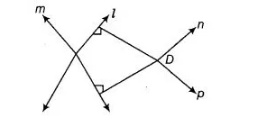# Prove that two lines that are respectively`
Question:

Prove that two lines that are respectively perpendicular to two intersecting lines intersect each other.

Solution:

Given Let lines l and m are two intersecting lines. Again, let n and p be another two lines which are perpendicular to the intersecting lines meet at point D.To prove Two lines n and p intersecting at a point.

Proof Suppose we consider lines n and p are not intersecting, then it means they are parallel to each other i.e., n || p …(i)

Since, lines n and pare perpendicular to m and l, respectively.

But from Eq. (i) n || p it implies that l || m.